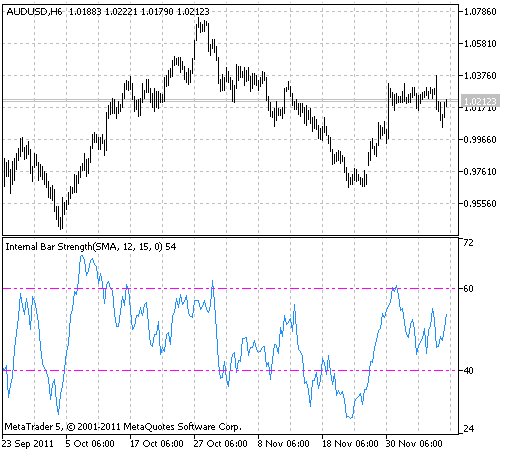Interesting script?
So post a link to it -
let others appraise it
You liked the script? Try it in the MetaTrader 5 terminal# IBS - indicator for MetaTrader 5

Rosh |
Views:
6298
Rating:
Published:
2012.02.03 15:17
Updated:
2023.03.29 13:44

Real author: Rosh

Internal Bar Strength (IBS) indicator is developed by Volker Knapp.

Calculation:

The indicator is calculated as a moving average of the bars inner strength values representing the following equation for each of them:

IBS = ((Close - Low) / (High - Low)) * 100%

Usage:

The period equal to five bars is usually used. The crossing of 60% level means the overbuying and the crossing of 40% level means overselling, and so they are the signals for selling and buying respectively.

Input parameters:

```//+-----------------------------------+
//| Indicator input parameters        |
//+-----------------------------------+
input Smooth_Method IMA_Method=MODE_SMA; // Smoothing method
input int ILength=12;                    // Smoothing depth
input int IPhase=15;                     // Smoothing parameter
input Applied_price_ IPC=PRICE_CLOSE_LOW;// Applied price
input int Shift=0;                       // Horizontal shift of the indicator in bars```

This indicator allows to change smoothing method:

1. SMA - simple moving average;
2. EMA - exponential moving average;
3. SMMA - smoothed moving average;
4. LWMA - linear weighted moving average;
5. JJMA - JMA adaptive average;
6. JurX - ultralinear smoothing;
7. ParMA - parabolic smoothing;
8. T3 - Tillson's multiple exponential smoothing;
9. VIDYA - smoothing with the use of Tushar Chande's algorithm;
10. AMA - smoothing with the use of Perry Kaufman's algorithm.

It should be noted that Phase type parameters for different smoothing algorithms have completely different meaning. For JMA it is an external Phase variable changing from -100 to +100. For T3 it is a smoothing ratio multiplied by 100 for better visualization, for VIDYA it is a CMO oscillator period and for AMA it is a slow EMA period. In other algorithms these parameters do not affect smoothing. For AMA fast EMA period is a fixed value and is equal to 2 by default. The ratio of raising to the power is also equal to 2 for AMA.

The indicator uses SmoothAlgorithms.mqh library classes (must be copied to the terminal_data_folder\MQL5\Include). The use of the classes was thoroughly described in the article "Averaging Price Series for Intermediate Calculations Without Using Additional Buffers".

This indicator was first implemented in MQL4 and published in CodeBase 10.10.2008.Translated from Russian by MetaQuotes Ltd.
Original code: https://www.mql5.com/ru/code/749X2MA_KLx3_Cloud

Keltner Channel displayed as colored background.FisherTransform_HTF_Signal

FisherTransform_HTF_Signal shows a trend direction as a graphical object with colored trend indication and gives alerts or audio signals in case of a trend change.Ultimate_Oscillator

The Ultimate Oscillator by Larry Williams is calculated as weighted value of three Stochastic, indicators, calculated for fast, middle and short periods.TrendManager

Visual trend indicator that shows the direction and power of the current price movement.